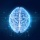Home
IT Knowledge
Inspiration
Languages
EN

# Java - replace first character in string

0 points
Created by:Zachariah
238

In this article, we would like to show you how to replace the first character in string in Java.

Quick solution:

``````String text = "ABC";
String replacement = "x";
String result = replacement + text.substring(1);

System.out.println(result);  // xBC``````

or:

``````String text = "ABC";
String replacement = "x";
String result = replacement.concat(text.substring(1));

System.out.println(result);  // xBC``````

or:

``````String text = "ABC";
String replacement = "x";
String result = text.replaceAll("^.", replacement);

System.out.println(result);  // xBC``````

## 1. Practical example using String `substring()`

### 1.1 With `+` operator

In this example, we remove last character from the original string and add the replacement at the beginning.

``````public class Example {

public static void main(String[] args) {
String text = "ABC";
String replacement = "x";
String result = replacement + text.substring(1);

System.out.println(result);  // xBC
}
}``````

### 1.2 With `concat()` method

This approach is equivalent to the above one. Instead of `+` operator we concatenate the strings using `concat()` method.

``````public class Example {

public static void main(String[] args) {
String text = "ABC";
String replacement = "x";
String result = replacement.concat(text.substring(1));

System.out.println(result);  // xBC
}
}``````

## 2. Practical example using String `replaceAll()` with regex pattern

In this example, we use string `replaceAll()` with `"^."` regex to replace the first character in the `text` string.

Regex explanation:

• `^` - matches the beginning of the string,
• `.` - matches any character except linebreaks.
``````public class Example {

public static void main(String[] args) {
String text = "ABC";
String replacement = "x";
String result = text.replaceAll("^.", replacement);

System.out.println(result);  // xBC
}
}``````

Note:

Regular expressions are slower than `substring()` method.